# The Momentum Indicator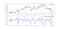EURUSD in the first panel with the 3-period Momentum Indicator in the second panel. (Image by Author)
`def momentum_indicator(Data, what, where, lookback):     for i in range(len(Data)):     Data[i, where] = Data[i, what] / Data[i - lookback, what] * 100        return Data# The Data variable is the OHLC array# The what variable is the closing price column# The where variable is where to put the indicator# The lookback variable is the subtraction range`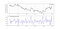USDCHF in the first panel with the 3-period Momentum Indicator in the second panel. (Image by Author)

# The Concept of Correlation

`def auto_correlation(Data, first_data, second_data, shift_degree, lookback, where):        new_array = shift(Data[:, first_data], shift_degree, cval = 0)    new_array = np.reshape(new_array, (-1, 1))        Data = np.concatenate((Data, new_array), axis = 1)    Data = adder(Data, 20)        for i in range(len(Data)):                try:            Data[i, where] = pearsonr(Data[i - lookback + 1:i + 1, first_data], Data[i - lookback + 1:i + 1, second_data])                      except ValueError:            pass        return Data`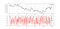USDCHF in the first panel with the 3-period Autocorrelation in the second panel. (Image by Author)

# The K’s Momentum Indicator — KMI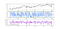EURUSD in the first panel, the 3-period Autocorrelation in the second panel, and the 3-period Momentum Indicator in the third panel. (Image by Author)

# Back-testing the Strategy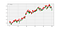Signal chart on the EURUSD following the RMI strategy. (Image by Author)
`def signal(Data, auto_corr, moment, buy, sell):        for i in range(len(Data)):                    if Data[i, auto_corr] > upper_barrier and Data[i, moment] < Data[i - lookback, moment]:            Data[i, buy] = 1if Data[i, auto_corr] > upper_barrier and Data[i, moment] > Data[i - lookback, moment]:            Data[i, sell] = -1`

# A Word on Risk Management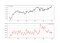EURUSD hourly data with the 50-period Exponential Average True Range. (Image by Author).

Written by